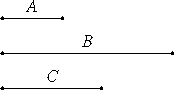# Proposition 30

If an odd number measures an even number, then it also measures half of it.

Let the odd number A measure the even number B.

I say that it also measures the half of it.Since A measures B, let it measure it according to C.

I say that C is not odd.

IX.23

If possible, let it be so. Then, since A measures B according to C, therefore A multiplied by C makes B. Therefore B is made up of odd numbers the multitude of which is odd. Therefore B is odd, which is absurd, for by hypothesis it is even. Therefore C is not odd, therefore C is even.

Thus A measures B an even number of times. For this reason then it also measures the half of it.

Therefore, if an odd number measures an even number, then it also measures half of it.

Q.E.D.

## Guide

This proposition is used in the next one.